# [Paper] MEON: End-to-End Blind IQA (Image Quality Assessment)

## Outperforms IQA-CNN, DeepIQA With Smaller Model Size

In this paper, “End-to-End Blind Image Quality Assessment Using Deep Neural Networks” (MEON), by University of Waterloo, and Harbin Institute of Technology, is presented. I read this because my colleague introduces this paper when I study about IQA. In this paper:

• A multi-task end-to-end optimized deep neural network (MEON) is proposed. It consists of two sub-networks — a distortion identification network and a quality prediction network — sharing the early layers.
• First, a distortion type identification sub-network (Sub-Network I) is trained.
• Then, starting from the pretrained early layers and the outputs of the first sub-network, a quality prediction sub-network (Sub-Network II) is trained.

This is a paper in 2018 TIP with over 100 citations where TIP has with high impact factor of 6.79. (Sik-Ho Tsang @ Medium)

# Outline

1. MEON: Network Architecture
2. MEON: Training and Testing
3. Ablation Study
4. Experimental Results

# 1. MEON: Network Architecture

## 1.1. Input and Subtasks

• MEON takes a raw image of 256 × 256 × 3 as input and predict its perceptual quality score.
• MEON consists of two subtasks accomplished by two sub-networks. Sub-network I aims to identify the distortion type in the form of a probability vector, which indicates the likelihood of each distortion and is fed as partial input to Sub-network II, whose goal is to predict the image quality.

## 1.2. GDN as Activation Function

• Generalized Divisive Normalization (GDN) is used as activation function as shown above. It has been previously demonstrated to work well in density estimation  and image compression .
• Specifically, given an S-dimensional linear convolutional activation
x(m,n) = [x1(m,n), · · · , xS(m,n)]T at spatial location (m,n), the GDN transform is defined as:
• where y(m,n) = [y1(m,n), · · · , yS(m,n)]T is the normalized activation vector. The weight matrix γ and the bias vector β are parameters in GDN to be optimized. Both of them are confined to [0,+∞).
• GDN is proven to be preserves better information than ReLU.
• On the other hand, GDN is different from BN in many ways. GDN offers high nonlinearities especially when it is cascaded in multiple stages.
• Compared with Local Response Normalization (LRN) used in AlexNet, LRN becomes a special case of GDN.
• (If interested, please feel free to read the paper about GDN.)

## 1.3. Shared Layers

• First, feed X(k), a set of k images, to the shared layers, which are responsible for transforming raw image pixels into perceptually meaningful and distortion relevant feature representations. It consists of four stages of convolution, GDN, and maxpooling.
• The spatial size is reduced by a factor of 4 after each stage via convolution with a stride of 2 (or without padding), and 2 × 2 maxpooling.

A 256 × 256 × 3 raw image is represented by a 64-dimensional feature vector.

## 1.4. Distortion Type Identification Sub-Network (Sub-Network I)

• On top of the shared layers, Sub-network I appends two fully connected layers with an intermediate GDN transform to increase nonlinearity.
• The softmax function to encode the range to [0, 1] which indicates the probability of each distortion type ˆp(k).
• This ˆp(k) is the quantity fed to sub-network II.
• To train Subtask I, the empirical cross entropy loss is used:
• where w1 are the weights for sub-task I.

## 1.5. Quality Prediction Sub-Network (Sub-Network II)

• Sub-network II takes the shared convolutional features and the estimated probability vector ˆp(k) from Sub-network I as inputs.
• It predicts the perceptual quality of X(k) in the form of a scalar value ˆ q(k).
• Two fully connected layers are used to produce a score vector s(k).
• Then, a fusion layer that combines ˆp(k) and s(k) to yield an overall quality score:
• A probability weighted summation as a simple implementation of g, i.e.:
• For subtask II, l1-norm as the empirical loss function is used:
• Therefore, the overall loss is:

# 2. MEON: Training and Testing

## 2.1. Training

• MEON tackles this problem by dividing the training into two steps: pre-training and joint optimization.
• At the pre-training step, the loss function in Subtask I is minimized.
• At the joint optimization step, the overall loss function is minimized.
• where w2 are the weights for sub-task II.

## 2.2. Testing

• 256 × 256 × 3 sub-images are extracted from a single image with a stride of U.
• The final distortion type is computed by the majority vote among all predicted distortion types of the extracted sub-images.
• Similarly, the final quality score is obtained by simply averaging all predicted scores.

# 3. Ablation Study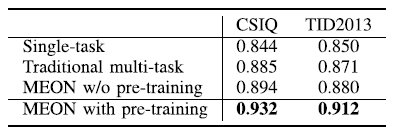Median SRCC Across 1000 Sessions on TID2013
• First, train Sub-network II with random initializations as a simple single-task baseline.
• Then, train the the traditional multi-task learning framework by directly producing an overall quality score.
• Finally, train MEON without and with pre-training.

It can be seen that the MEON framework and the pre-training mechanism are keys to the success of MEON.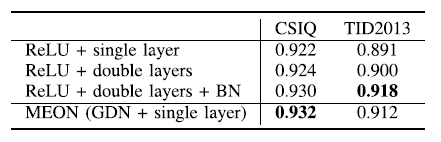Median SRCC Across 1000 Sessions on TID2013
• First, replace all GDN with ReLU as a baseline network.
• Then double all convolutional and fully connected layers in both Sub-networks I and II with ReLU as a deeper network.
• Afterwards, batch normalization (BN) is used on top of it.

We see that simply replacing GDN with ReLU leads to inferior performance.

GDN is an effective way to reduce model complexity without sacrificing performance.

# 4. Experimental Results

## 4.1. Performance on CSIQ and TID2013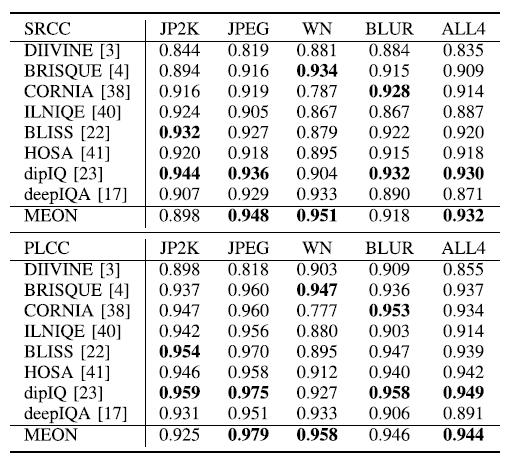Median SRCC and PLCC results Across 1000 Sessions on CSIQ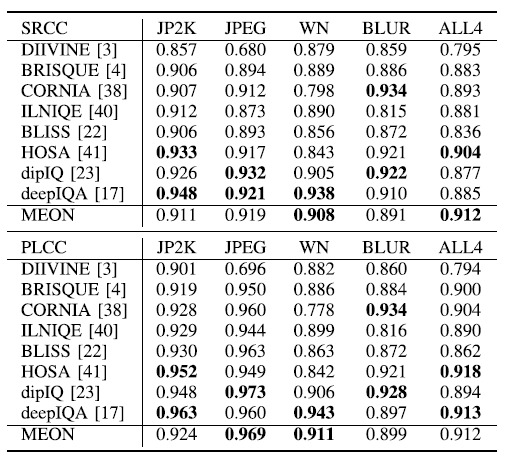Median SRCC and PLCC results Across 1000 Sessions on TID2013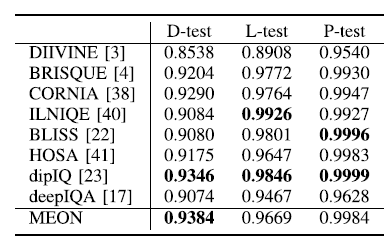D-Test, L-Test, and P-Test on Waterloo Exploration Dataset
• MEON achieves state-of-the-art performance on all three databases.
• MEON significantly outperforms DIIVINE, an improved version of BIQI with more advanced NSS. The performance improvement is largely due to the jointly end-to-end optimization.
• D-test quantifies the ability of a BIQA model to discriminate pristine from distorted images.
• MEON performs the best in D-test on the Exploration database, which is no surprise because a finer-grained version of D-test is performed through Subtask I.

The performance improvement is obtained because

1) the proposed novel learning framework has the quality prediction subtask regularized by the distortion identification subtask;

2) images instead of patches are used as inputs to reduce the label noise;

3) the pre-training step helps to achieve the better local minimum.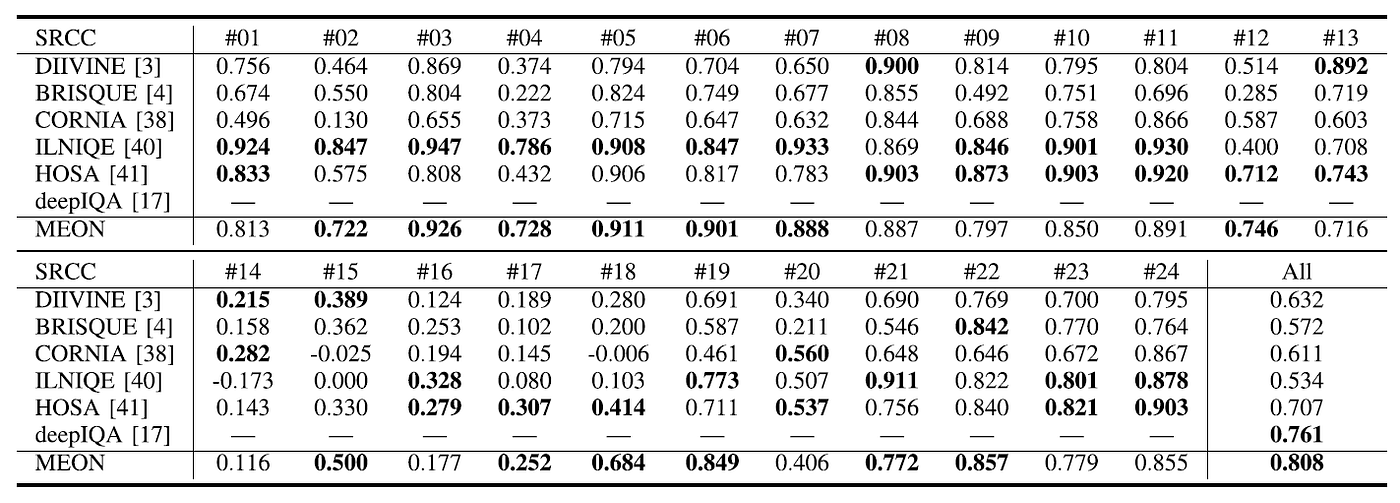Median SRCC and PLCC results Across 10 Sessions on Full TID2013

## 4.2. Model Size

• MEON has a small model size compared with other SOTA NR-IQA approaches such as IQA-CNN (Kang14) and DeepIQA, and has a comparable model size with IQA-CNN++ (Kang15).

## Reference

[2018 TIP] [MEON]
End-to-End Blind Image Quality Assessment Using Deep Neural Networks

## Image Quality Assessment (IQA)

[IQA-CNN] [IQA-CNN++] [DeepSim] [DeepIQA] [MEON]

--

--